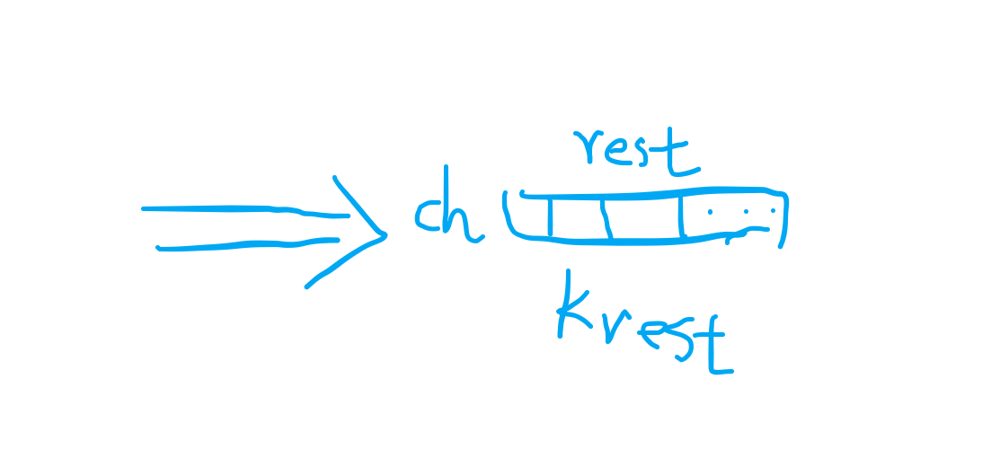By Long Luo

# Greedy

As we want to minimize the lexicographical order of the constructed string, let’s start from the beginning of the string, select the smallest letter that satisfies the requirements each time, then we get the final answer.

Suppose we are currently constructed to a certain position, as the picture below shows.Including this position, there are still $n_{rest}$ left to fill in, the remaining sum of these rest positions is $k_{rest}$.

If we put a letter $\textit{ch}$, and so the remaining $n_{rest} - 1$ positions with the sum is $k_{rest}$ must satisfy:

$1 \times (n_{rest}-1) \leq k_{rest}-ch \leq 26 \times (n_{rest}-1)$

can be:

$k_{rest}-26 \times (n_{rest}-1 ) \leq ch \leq k_{rest}-1 \times (n_{rest}-1)$

So $\textit{ch}$

1. $k_{rest} - 26 \times (n_{rest}-1) \leq 0$, we choose the character $a$;
2. $k_{rest} - 26 \times (n_{rest}-1) \gt 0$, we choose the character corresponding to this value.

Let’s write the code:

## Analysis

• Time Complexity: $O(n)$
• Space Complexity: $O(n)$

All suggestions are welcome.
If you have any query or suggestion please comment below.
Please upvote👍 if you like💗 it. Thank you:-)

Explore More Leetcode Solutions. 😉😃💗